# fourier transform properties

TheFourier transformof a real, continuous-time signal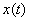is a complex-valued function defined by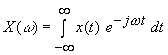where w is a real variable (frequency, in radians/second) and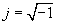.

A plot of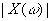vs w is called the magnitude spectrum of, and a plot of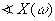vs wis called the phase spectrum of. These plots, particularly the magnitude spectrum, provide a picture of the frequency composition of the signal. For a real signal, the magnitude spectrum is an even function of frequency,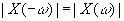. The phase spectrum will be plotted for angles in the principle range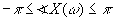, and the choice between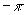and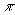will be made so that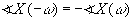, for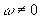. That is, the phase spectrum will be shown as an odd function of frequency, except that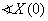might not be zero.

For a number of signals of interest, the Fourier transform integral does not converge in the usual sense of elementary calculus. Some of these signals can be treated in a consistent fashion by admitting Fourier transforms that contain impulses. For example, if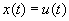, the unit-step signal, then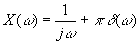For such a Fourier transform, we treat impulse components as separate in computing the magnitude spectrum since an impulse is zero at all values of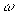but one, though admittedly something very special happens at that one point. Thus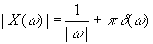In plotting the magnitude spectrum, we indicate the impulse term using an arrow. For the phase spectrum display, we ignore any impulse term, which contributes angle, the angle of the “area” of the impulse, at only one value of. Thus for the unit-step signal the phase spectrum is given by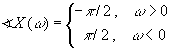The applet below illustrates properties of the magnitude and phase spectra of signals, and the effect on the spectra of typical operations on signals. Select a signal from the provided signals, and the corresponding magnitude and phase spectra will be displayed. Then select an operation and the resulting signal and its spectra are displayed.

Note that impulses are shown as arrows, but the area is not indicated. Also, amplitude scaling an impulse should be interpreted as area scaling. Finally, the cosine pulse is chosen so that the pulse begins and ends at a zero crossing of the cosine.

x(t)

X(w)

## unit impulse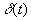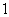unit step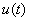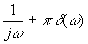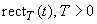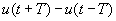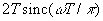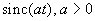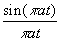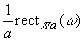exponential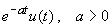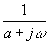t-exponential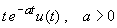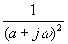tent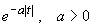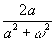Gaussian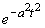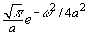cosine pulse,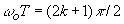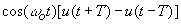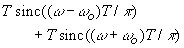For each signal, you can select an operation and the effects of the operation on the signal and its spectra are displayed. The available operations are described in the table below.

## Operation on x(t)

Resulting signal y(t)

Y(w)

amplitude scale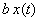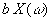time shift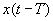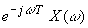time scale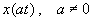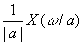time reverse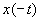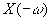derivative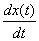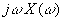running integral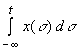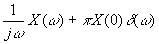In justifying the spectra corresponding to an operation, you might need to apply standard properties of impulses, in particular,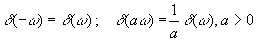and, if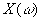is an ordinary function that is continuous at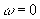,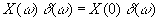For example, the time shifted unit-step signal,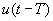, corresponds to the Fourier transform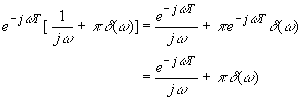Furthermore, derivatives of discontinuous signals must be interpreted in the generalized sense. For example, the derivative of the unit step is the unit impulse, and the corresponding transform operation gives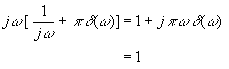(An impulse of zero area is interpreted as no impulse.)

The derivative of an impulse is a “doublet.” This generalized signal is shown as an up/down arrow, but the mathematical properties of doublets are beyond our scope. (Note that the running integral operation on a signal with an ordinary Fourier transform typically yields a signal that has an impulsive Fourier transform. Also, the running integral of a unit step is a unit ramp, another signal whose transform involves a doublet.)

Exercises

• Time scaling by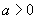leaves a unit-step function unchanged. Verify this mathematically by showing that the Fourier transform of the step is unchanged, using the time scaling property.

• Verify the displayed magnitude spectrum for the time derivative of the exponential signal. Note the product rule gives the generalized derivative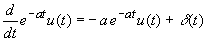·        How do you distinguish between the time reversal and the amplitude reversal (amplitude scale by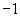) of a signal based on the resulting spectra? (For example, beginning with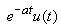consider the spectra of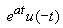and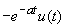.)

·        What is the area of the impulse in the Fourier transform of the running integral of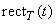?

·        Mathematically justify the form of the magnitude spectrum of the derivative of.